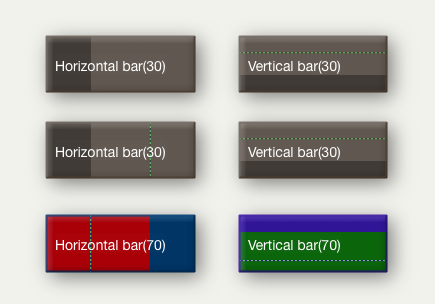# Pattern: bar(30)Note that this pattern cannot be set via the Interior Inspector, use the Plot Inspector. The pattern requires configuration via its arguments. This pattern can be used in shaped notes.

bar(value,[min],[max][,target])

This draws as a horizontal 'progress bar', using \$Color and \$AccentColor. The 'progress' block is drawn in \$AccentColor. These colours are set via the Interior Inspector.

The arguments work this way:

• value. If used alone it is a percentage, otherwise it is a value between min and max with the bar being set using value as a percentage of max-min (which must thus be specified). Thus `bar(25)` is the same as `bar(37.5,25,75)`.
• min & max. Numerical minimum and maximum values for computing the degree of 'progress' represented in \$AccentColor by value. Both arguments may be negative numbers; if min is not supplied, a value of 0 is assumed and negative attribute values are plotted as if zero.
• target. The target represents a nominal or desired result. For example, if normally writing between 0 and 4000 words on any given day, it might be useful to set a target at 1500 words/day. If setting a target, a min and max must also be set. The target line is drawn in a dashed link alternating \$PlotColor and either \$Color or \$AccentColor) whichever is the current background colour in the position of the target line.

The arguments in the brackets may be numbers or expressions that can be evaluated as numbers. As \$Pattern is a string argument, remember to enclose the statement in quotes so the program coerces the output to a string. If a note's \$Width is 3.0, then

`\$Pattern="bar(15*\$Width)"`

is the same as writing

`\$Pattern="bar(45)"`

Note that if the value to be use is an action code variable, a slightly different encoding is needed to ensure the value and not the name of the variable is used. Here, our progress percentage is in a variable 'vPercent':

`\$Pattern="bar("+vPercent+")"`

or more accurately:

`\$Pattern="'bar('+vPercent+')'"`

… though both forms seem to work - use which ever feels more intuitive.

If the pattern is written without arguments or brackets, it evaluates as if at 50%, e.g. `bar()` and `bar ` are the same as `bar(50)`.

The same pattern can be rendered on a vertical access using the vbar() pattern.